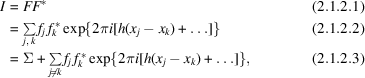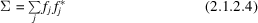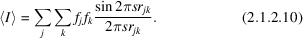International
Tables for
Crystallography
Volume B
Reciprocal space
Edited by U. Shmueli

International Tables for Crystallography (2010). Vol. B, ch. 2.1, pp. 195-196   | 1 | 2 |

Section 2.1.2. The average intensity of general reflections

U. Shmuelia* and A. J. C. Wilsonb

aSchool of Chemistry, Tel Aviv University, Tel Aviv 69 978, Israel, and bSt John's College, Cambridge, England
Correspondence e-mail:  ushmueli@post.tau.ac.il

2.1.2. The average intensity of general reflections

| top | pdf |

2.1.2.1. Mathematical background

| top | pdf |

The process may be illustrated by evaluating, or attempting to evaluate, the average intensity of reflection by the three processes. The intensity of reflection is given by multiplying equation (2.1.1.1)by its complex conjugate:whereis the sum of the squares of the moduli of the atomic scattering factors. Wilson (1942) argued, without detailed calculation, that the average value of the exponential term would be zero and hence thatAveraging equation (2.1.2.3)for hkl fixed, xyz ranging uniformly over the unit cell – the first process described above – gives this result identically, without complication or approximation. Ordinarily the second process cannot be carried out. We can, however, postulate a special case in which it is possible. We take a homoatomic structure and before averaging we correct the f's for temperature effects and the fall-off with, so thatis the same for all the atoms and is independent of hkl. If the range of hkl over which the expression for I has to be averaged is taken as a parallelepiped in reciprocal space with h ranging from −H to +H, k from −K to +K, l from −L to +L, equation (2.1.2.2)can be factorized into the product of the sums of three geometrical progressions. Algebraic manipulation then easily leads towhere,and. The terms with j = k give Σ, but the remaining terms are not zero. Because of the periodic nature of the trigonometric terms, the effective coordinate differences are never greater than 0.5 and in a structure of any complexity there will be many much less than 0.5. For HKL = 000, in fact,becomes the square of the modulus of the sum of the atomic scattering factors,whereand not the sum of the squares of their moduli; for larger HKL,rapidly decreases to Σ and then oscillates about that value. Wilson (1949, especially Section 2.1.1) suggested that the regions of averaging should be chosen so that at least one index of every reflection isifis to be identified with Σ, and this has proven to be a useful rule-of-thumb.

The third process of averaging replaces the sum over integral values of the indices by an integration over continuous values, the appropriate values of the limits in this example beingto. The effect is to replace the sines in the denominators, but not in the numerators, of equation (2.1.2.6)by their arguments, and this is equivalent to the approximationin the denominators only. This is a good approximation for atoms close together in the structure and thus giving the largest terms in the sums in equation (2.1.2.6), and gives the correct sign and order of magnitude even for x having its maximum value of π/2.

2.1.2.2. Physical background

| top | pdf |

The preceding section has used mathematical arguments. From a physical point of view, the radiation diffracted by atoms that are resolved will interfere destructively, so that the resulting intensity will be the sum of the intensities diffracted by individual atoms, whereas that from completely unresolved atoms will interfere constructively, so that amplitudes rather than intensities add. In intermediate cases there will be partial constructive interference. Resolution in accordance with the Rayleigh (1879) criterion requires thatshould be greater than half the reciprocal of the minimum interatomic distance in the crystal (Wilson, 1979); full resolution requires a substantial multiple of this. This criterion is essentially equivalent to that proposed from the study of a special case of the second process in the preceding section.

2.1.2.3. An approximation for organic compounds

| top | pdf |

In organic compounds there are very many interatomic distances of about 1.5 or 1.4 Å. Adoption of the preceding criterion would mean that the inner portion of the region of reciprocal space accessible by the use of copper Kα radiation is not within the sphere of intensity statistics based on fixed-index (first process) averaging. No substantial results are available for fixed-parameter (second process) averaging, and very few from the approximation to it (third process).

To the extent to which the third process is acceptable, an approximation to the variation ofwithis obtainable. The exponent in equation (2.1.2.2)can be written aswhere s is the radial distance in reciprocal space,is the distance from the jth to the kth atom andis the angle between the vectors s and r. Averaging over a sphere of radius s, withtreated as the colatitude, givesThis is the familiar Debye expression. It has the correct limits for s zero and s large, and is in accord with the argument from resolution.

2.1.2.4. Effect of centring

| top | pdf |

In the preceding discussion there has been a tacit assumption that the lattice is primitive. A centred crystal can always be referred to a primitive lattice and if this is done no change is required. If the centred lattice is retained, many reflections are identically zero and the intensity of the nonzero reflections is enhanced by a factor of two (I and C lattices) or four (F lattice), so that the average intensity of all the reflections, zero and nonzero taken together, is unchanged.

Other symmetry elements affect only zones and rows of reflections, and so do not affect the general average when the total number of reflections is large. Their effect on zones and rows is discussed in Section 2.1.3.

References

Rayleigh, Lord (1879). Investigations in optics with special reference to the spectroscope. Philos. Mag. 8, 261–274.
Wilson, A. J. C. (1942). Determination of absolute from relative intensity data. Nature (London), 150, 151–152.
Wilson, A. J. C. (1949). The probability distribution of X-ray intensities. Acta Cryst. 2, 318–320.
Wilson, A. J. C. (1979). Problems of resolution and bias in the experimental determination of the electron density and other densities in crystals. Acta Cryst. A35, 122–130.